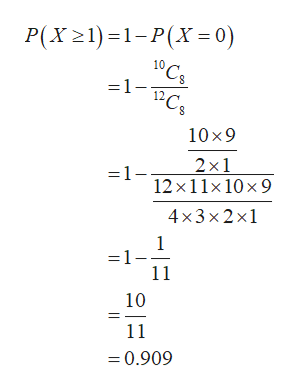# Whenever Suzan sees a bag of marbles, she grabs a handful at random. She has seen a bag containing four red marbles, two green ones, five white ones, and one purple one. She grabs eight of them. Find the probability of the following event, expressing it as a fraction in lowest terms.She has at least one green one.

Question
41 views

Whenever Suzan sees a bag of marbles, she grabs a handful at random. She has seen a bag containing four red marbles, two green ones, five white ones, and one purple one. She grabs eight of them. Find the probability of the following event, expressing it as a fraction in lowest terms.

She has at least one green one.
check_circle

Step 1

Total number of marbles = 4+2+5+1

=12

Total number of marbles without green = 4+5+1

= 10

Total number of green marbles = 2

Step 2

Let X is random variable that represents the number of green marbles.

If she grabs eight of them tha...help_outlineImage TranscriptioncloseP(X21)1-P(X= 0) 10 'Cs =1- 10 x9 2x1 12x11x 10x 9 =1- 4x3x2x1 1 =1- 11 10 11 =0.909 fullscreen

### Want to see the full answer?

See Solution

#### Want to see this answer and more?

Solutions are written by subject experts who are available 24/7. Questions are typically answered within 1 hour.*

See Solution
*Response times may vary by subject and question.
Tagged in

### Basic Probability